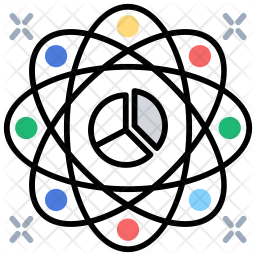cancel
Showing results for
Did you mean:Frequent Visitor

## Modify Filter Based on Date

Hi, community,

I develop reports on projects' progress by cycle.

The data is a combination of different sources and looks like this:

 Cycle Title Start Finish Cycle Currency Id 2020-01 7/01/2020 17/02/2020 1 2020-02 18/02/2020 30/03/2020 2 2020-03 31/03/2020 11/05/2020 3 2020-04 12/05/2020 22/06/2020 4 2020-05 23/06/2020 3/08/2020 5 2020-06 11/08/2020 21/09/2020 6 2020-07 22/09/2020 2/11/2020 7 2020-08 3/11/2020 14/12/2020 8

There is a week break after cycle 2020-05 and there is a Christmas break with no cycles.

I use currency ids to filter content based on the cycle. I use a page filter to do this.

When it comes to the break weeks I need to display the content from the previous cycle.

More details:

1. Every cycle is 6 weeks long

2. The first cycle of the year starts on the first Tuesday of the year

3. Mid-year break week occurs after the 04th or 05th cycle of the year. There is a break until the first Tuesday of the New Year from the end of the last cycle.

4. Cycle Currency Id is generated based on the current date. If today falls between the cycle dates then it gives cycle Id 1, and so on. So the ids change based on within which cycle we are now.

5. I can't add week breaks' information directly into the data source.

Thanks

Evan

1 ACCEPTED SOLUTIONCommunity Support

Hi  @EBGAL ,

Here are the steps you can follow：

1. Create calculated column.

``````Datediff =
var _lastfinish=CALCULATE(MAX('Table'[Finish]),FILTER(ALL('Table'),[Cycle Currency Id]=EARLIER([Cycle Currency Id])-1))
return
IF([Cycle Currency Id]=1,1,DATEDIFF(_lastfinish,[Start],DAY))``````

2. Create measure.

``````Measure =
var _select=SELECTEDVALUE('Table'[Cycle Currency Id])
var _gap=CALCULATE(MAX('Table'[Cycle Currency Id]),FILTER(ALL('Table'),[Datediff]>1))-1
return
IF(
ISFILTERED('Table'[Cycle Currency Id]),
MAX('Table'[Start])&"-"&MAX('Table'[Finish]),
MAXX(FILTER(ALL('Table'),[Cycle Currency Id]=_gap),[Start])&"-"&MAXX(FILTER(ALL('Table'),[Cycle Currency Id]=_gap),[Finish]))``````

3. Put [cycle currency ID] into slicer and [measure] into card.

4. Result:

If it is not selected, it will be displayed as the previous cycle of the rest week.When selecting, display the current cycle.Best Regards,

Liu Yang

If this post helps, then please consider Accept it as the solution to help the other members find it more quickly.

3 REPLIES 3Community Support

Hi  @EBGAL ,

Here are the steps you can follow：

1. Create calculated column.

``````Datediff =
var _lastfinish=CALCULATE(MAX('Table'[Finish]),FILTER(ALL('Table'),[Cycle Currency Id]=EARLIER([Cycle Currency Id])-1))
return
IF([Cycle Currency Id]=1,1,DATEDIFF(_lastfinish,[Start],DAY))``````

2. Create measure.

``````Measure =
var _select=SELECTEDVALUE('Table'[Cycle Currency Id])
var _gap=CALCULATE(MAX('Table'[Cycle Currency Id]),FILTER(ALL('Table'),[Datediff]>1))-1
return
IF(
ISFILTERED('Table'[Cycle Currency Id]),
MAX('Table'[Start])&"-"&MAX('Table'[Finish]),
MAXX(FILTER(ALL('Table'),[Cycle Currency Id]=_gap),[Start])&"-"&MAXX(FILTER(ALL('Table'),[Cycle Currency Id]=_gap),[Finish]))``````

3. Put [cycle currency ID] into slicer and [measure] into card.

4. Result:

If it is not selected, it will be displayed as the previous cycle of the rest week.When selecting, display the current cycle.Best Regards,

Liu Yang

If this post helps, then please consider Accept it as the solution to help the other members find it more quickly.Frequent Visitor

Hi, @v-yangliu-msft ,

This works.

I managed to achieve the same in Power Query by adding the column StartNextCycle which is lagged Start column.

Instead of generating Cycle Currency Id if it is between Start and Finish, I changed it to the condition if today is between Start and StartNextCycle.

The solution appeared simpler than I thought.

Thanks

EvanSuper User IV

@EBGAL , From where you are selecting date, based on we can suggest the formula

example

measure =
var _max = maxx(allselected('Date'), 'Date'[Date])
var _1 = calculate(countrows(Table), filter(Table, Table[Start date] <=_max && Table[Finish date] >=_max))
return
if(isblank(_1), calculate(countrows(Table), filter(Table, Table[Start date] <=_max-7 && Table[Finish date] >=_max-7)) , _1)

or

measure =
var _max = maxx(allselected('Date'), 'Date'[Date])
var _1 = calculate(countrows(Table), filter(Table, Table[Start date] <=_max && Table[Finish date] >=_max))
return
if(isblank(_1), calculate(countrows(Table), filter(all(Table), Table[Start date] <=_max-7 && Table[Finish date] >=_max-7)) , _1)

Proud to be a Super User!Announcements#### Welcome to the User Group Public Preview